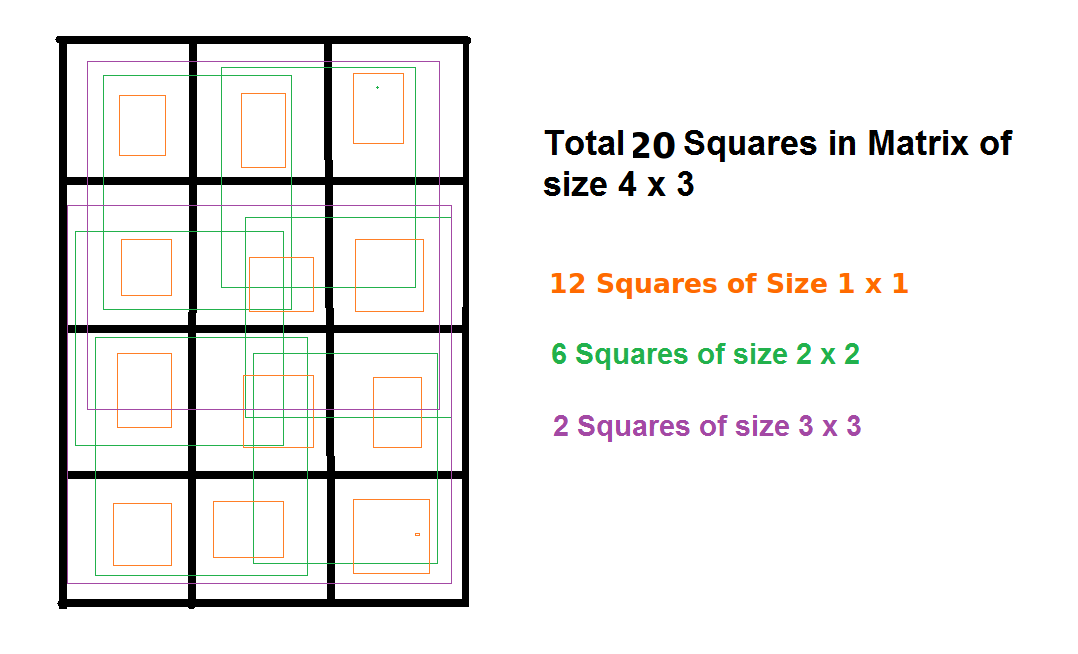# Sum of Area of all possible square inside a rectangle

• Difficulty Level : Medium
• Last Updated : 22 Jun, 2022

Given two integers L and B denoting the length and breadth of a rectangle respectively. The task is to calculate the sum of the area of all possible squares that comes into the rectangle.
Examples

```Input: L = 4, B = 3
Output: 54

Input:  L = 2, B = 5
Output: 26```

The idea is to observe the count of number of squares in a rectangleNow, the number of squares of side 1 will be 12 as there will be two cases one as squares of 1-unit sides along the horizontal(3) and second case as squares of 1-unit sides along the vertical(4). That gives us 3*4 = 12 squares.
When the side is 2 units, one case will be as squares of side of 2 units along only one place horizontally and second case as two places vertically. So the number of squares = 6
So we can deduce that,
Number of squares of size 1*1 will be L*B
Number of squares of size 2*2 will be (L-1)(B-1)
Therefore, the number of squares with sizewill be:

Number of square of size K = (L-K+1)*(B-K+1)

Therefore, area of total number of squares of size K will be:

Area of total number of square of size K = (L-K+1)*(B-K+1)*K*K

Below is the implementation of above idea:

## C++

 `// CPP program to calculate the sum of area` `// of all possible squares that comes` `// inside the rectangle`   `#include ` `using` `namespace` `std;`   `// Function to calculate the sum of area` `// of all possible squares that comes` `// inside the rectangle` `int` `calculateAreaSum(``int` `l, ``int` `b)` `{` `    ``int` `size = 1;` `    `  `    ``// Square with max size possible` `    ``int` `maxSize = min(l,b);` `    `  `    ``int` `totalArea = 0;` `    `  `    ``for``(``int` `i=1; i <= maxSize; i++)` `    ``{   ` `        ``// calculate total square of a given size` `        ``int` `totalSquares = (l-size+1)*(b-size+1);` `        `  `        ``// calculate area of squares of a` `        ``// particular size    ` `        ``int` `area = totalSquares*size*size;` `        `  `        ``// total area    ` `        ``totalArea += area;` `         `  `        ``// increment size    ` `        ``size++;` `    ``}` `    `  `    ``return` `totalArea;` `}`   `// Driver Code` `int` `main()` `{` `    ``int` `l = 4, b = 3;` `    `  `    ``cout<

## Java

 `// Java program to calculate the ` `// sum of area of all possible ` `// squares that comes inside` `// the rectangle` `class` `GFG ` `{` `// Function to calculate the ` `// sum of area of all possible ` `// squares that comes inside` `// the rectangle` `static` `int` `calculateAreaSum(``int` `l, ``int` `b)` `{` `    ``int` `size = ``1``;`   `    ``// Square with max size possible` `    ``int` `maxSize = Math.min(l, b);`   `    ``int` `totalArea = ``0``;`   `    ``for``(``int` `i = ``1``; i <= maxSize; i++)` `    ``{ ` `        ``// calculate total square ` `        ``// of a given size` `        ``int` `totalSquares = (l - size + ``1``) * ` `                           ``(b - size + ``1``);` `    `  `        ``// calculate area of squares ` `        ``// of a particular size ` `        ``int` `area = totalSquares *   ` `                    ``size * size;` `    `  `        ``// total area ` `        ``totalArea += area;` `        `  `        ``// increment size ` `        ``size++;` `    ``}`   `    ``return` `totalArea;` `}`   `// Driver Code` `public` `static` `void` `main(String[] args) ` `{` `    ``int` `l = ``4``, b = ``3``;` `    `  `    ``System.out.println(calculateAreaSum(l, b));` `}` `}`   `// This code is contributed ` `// by ChitraNayal`

## Python 3

 `# Python 3 program to calculate` `# the sum of area of all possible ` `# squares that comes inside ` `# the rectangle`   `# Function to calculate the ` `# sum of area of all possible` `# squares that comes inside` `# the rectangle` `def` `calculateAreaSum(l, b):` `    ``size ``=` `1` `    `  `    ``# Square with max size possible` `    ``maxSize ``=` `min``(l, b)` `    `  `    ``totalArea ``=` `0` `    `  `    ``for` `i ``in` `range``(``1``, maxSize ``+` `1` `):`   `        ``# calculate total square` `        ``# of a given size` `        ``totalSquares ``=` `((l ``-` `size ``+` `1``) ``*` `                        ``(b ``-` `size ``+` `1``))` `        `  `        ``# calculate area of squares ` `        ``# of a particular size ` `        ``area ``=` `(totalSquares ``*` `                ``size ``*` `size)` `        `  `        ``# total area ` `        ``totalArea ``+``=` `area` `        `  `        ``# increment size ` `        ``size ``+``=` `1` `        `  `    ``return` `totalArea`   `# Driver Code` `if` `__name__ ``=``=` `"__main__"``:` `    ``l ``=` `4` `    ``b ``=` `3` `    `  `    ``print``(calculateAreaSum(l,b))`   `# This code is contributed ` `# by ChitraNayal`

## C#

 `// C# program to calculate the ` `// sum of area of all possible ` `// squares that comes inside` `// the rectangle` `using` `System;`   `class` `GFG ` `{` `// Function to calculate the ` `// sum of area of all possible ` `// squares that comes inside ` `// the rectangle` `static` `int` `calculateAreaSum(``int` `l,` `                            ``int` `b)` `{` `    ``int` `size = 1;`   `    ``// Square with max size possible` `    ``int` `maxSize = Math.Min(l, b);`   `    ``int` `totalArea = 0;`   `    ``for``(``int` `i = 1; i <= maxSize; i++)` `    ``{ ` `        ``// calculate total square` `        ``// of a given size` `        ``int` `totalSquares = (l - size + 1) * ` `                           ``(b - size + 1);` `    `  `        ``// calculate area of squares ` `        ``// of a particular size ` `        ``int` `area = totalSquares *` `                   ``size * size;` `    `  `        ``// total area ` `        ``totalArea += area;` `        `  `        ``// increment size ` `        ``size++;` `    ``}` `    ``return` `totalArea;` `}`   `// Driver Code` `public` `static` `void` `Main() ` `{` `    ``int` `l = 4, b = 3;` `    `  `    ``Console.Write(calculateAreaSum(l,b));` `}` `}`   `// This code is contributed` `// by ChitraNayal`

## PHP

 ``

## Javascript

 ``

Output:

`54`

Time complexity: O(min(l,b))

space complexity: O(1)

My Personal Notes arrow_drop_up
Recommended Articles
Page :